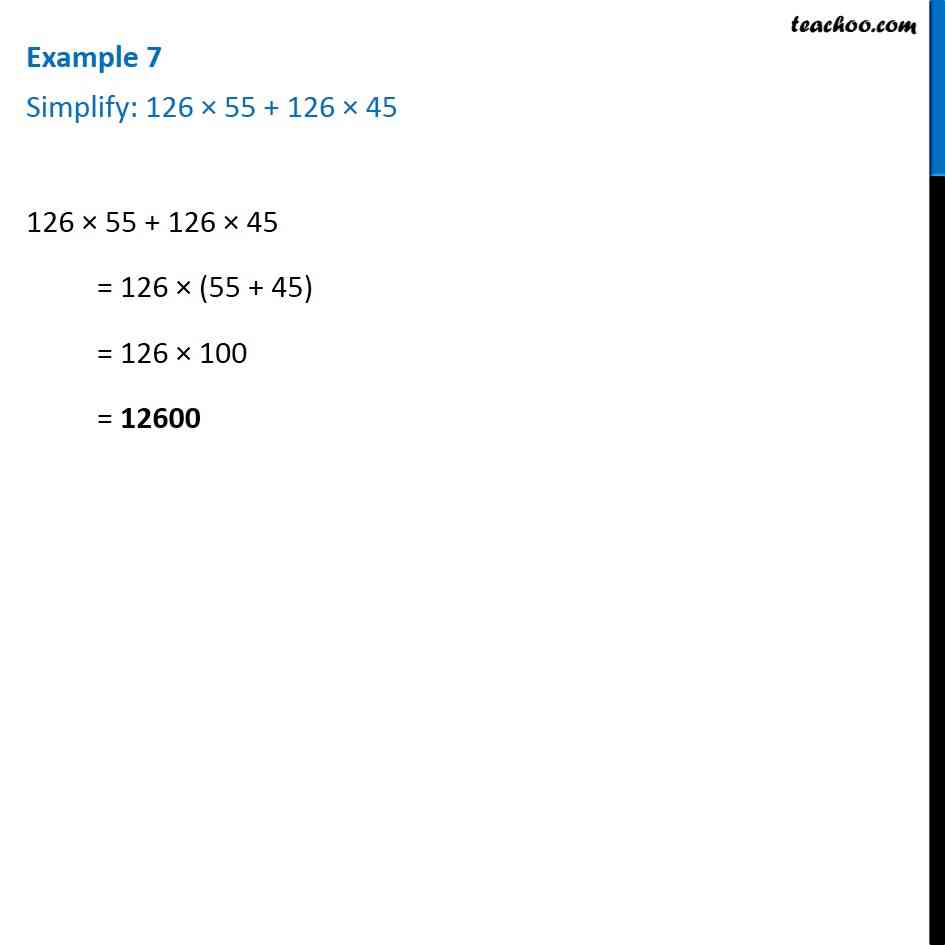1. Chapter 2 Class 6 Whole Numbers
2. Serial order wise
3. Examples

Transcript

Example 7 of Chapter 2 Class 6 Whole Numbers Simplify: 126 × 55 + 126 × 45 126 × 55 + 126 × 45 Taking 126 common = 126 × (55 + 45) = 126 × 100 = 12600

Examples